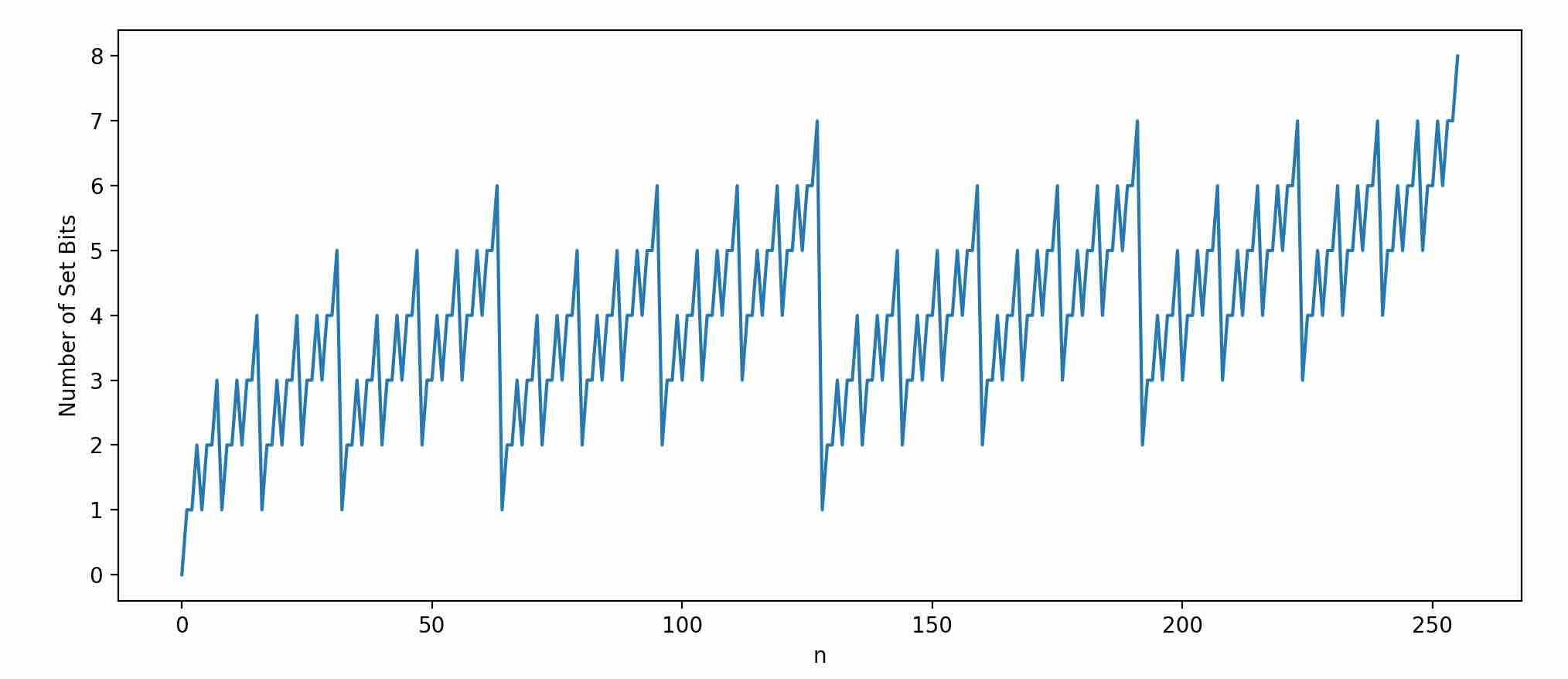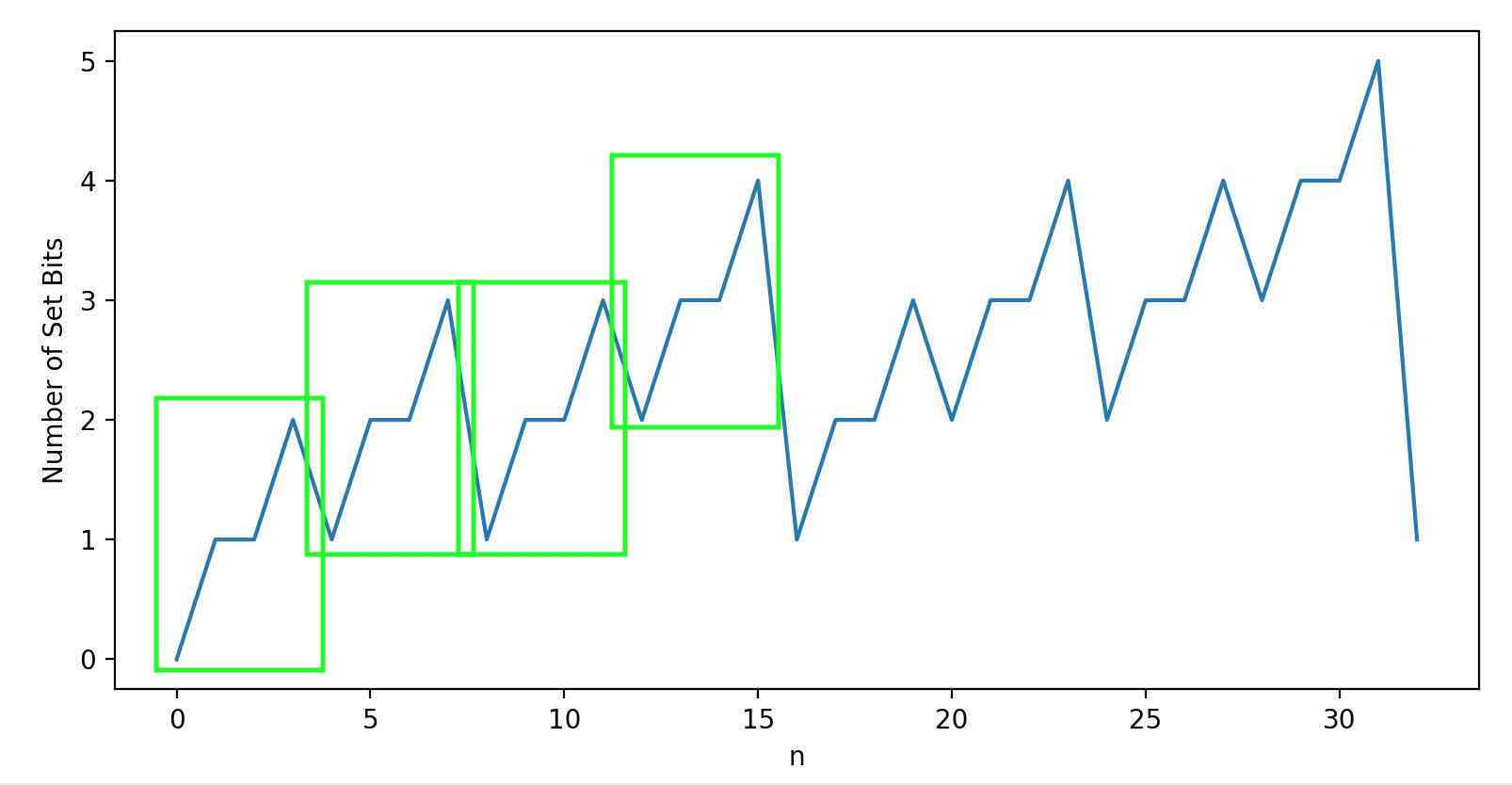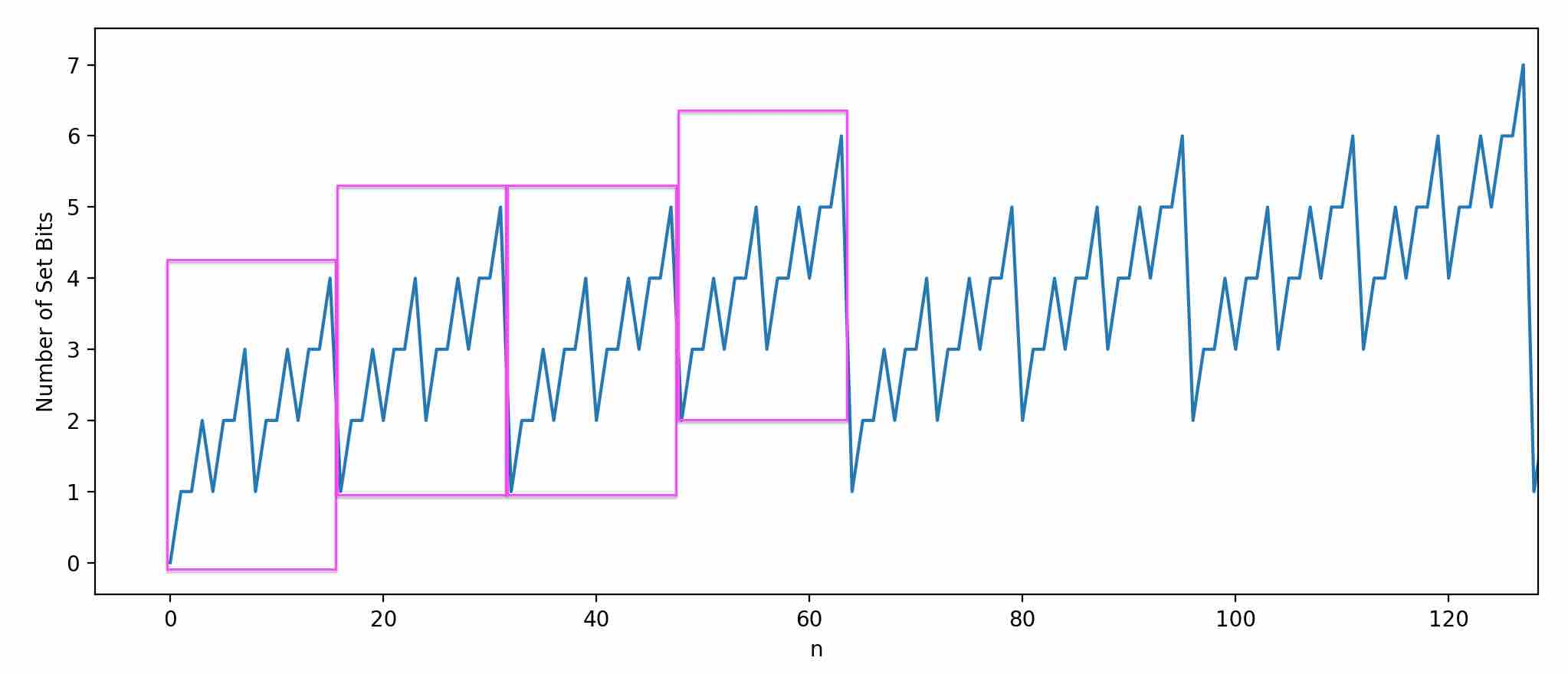# Population Count Pattern

Understanding why a concise, macro-defined array can be used as a lookup table for finding the number of set bits in a number.

# programming

2023-10-24

I was looking at Sean Anderson’s “Bit Twiddling Hacks” page. One method for counting set bits using a lookup table caught my attention.

Here’s a similar version, modified for completeness.

``````// https://graphics.stanford.edu/~seander/bithacks.html#CountBitsSetTable

#include <stdlib.h>
#include <stdio.h>
#include <stdint.h>

#define B2(n) n, n+1, n+1, n+2
#define B4(n) B2(n), B2(n+1), B2(n+1), B2(n+2)
#define B6(n) B4(n), B4(n+1), B4(n+1), B4(n+2)

static const uint8_t table = {
B6(0), B6(1), B6(1), B6(2)
};

#define BASE_10 10

int main(int argc, char **argv) {
uint64_t target = strtoull(argv, NULL, BASE_10);
uint8_t count = 0;
uint8_t *p = (uint8_t *)&target;

for (int i = 0; i < sizeof(p); i++) {
count += table[p[i]];
}

printf("Number of set bits in %llu is %u\n",
target, count);

return 0;
}``````

The program takes a number between `[0, 18446744073709551615]` and determines its population count, which is the number of bits that are set to 1.

Each 8-bit integer in the `table` array is the population count for the given index. The values can only go up to 8, since the indices range from `[0, 255]`.

The lookup table is still able to solve the population count for numbers greater than 255 because, for example, `popcount(255)` is the same thing as `popcount(15) + popcount(15)`.

``````┌─────────┬──────────────┐
│ BASE 10 │    BASE 2    │
├─────────┼──────────────┤
│ 255     │    1111 1111 │
│ 15      │    0000 1111 │
└─────────┴──────────────┘``````

Though `target` is an `unsigned long long`, it’s treated as an array of eight 8-bit values, which are used to index into the lookup table, and then summed up to get the final result.

This is probably not the absolute fastest way to find the population count, and there are even clever ways to use less memory, but the table is based on an interesting pattern that took me a little while to understand why it works.

Not too surprisingly, when binary numbers are enumerated, there’s a pattern of how many `1`‘s appear from one number to the next, though it’s not immediately apparent what that pattern is.

Graphing the number of set bits for every binary number helps to visualize the pattern.Zoom in closer and you can start to pinpoint what the pattern actually is.Within each green box there are four population counts, which basically go like this as `n` increases:

• Increase
• Stay the same
• Increase

Think of `n` as the first point within a box. How do the other points in the same box change, with respect to the first point, `n`?

They do this: `n+1, n+1, n+2`. With knowledge of this pattern, a lookup table of the first four pre-computed bit counts can be created.

``````#define B2(n) n, n+1, n+1, n+2

static const uint8_t table = {
B2(0)
};``````

Not too useful, but the same pattern can be applied to grow the array. Notice in the graph that the green boxes themselves also follow the same pattern: The first box begins lower than the others, the next two boxes stay the same, and the final box sits higher than the others.

It’s the same pattern, so it can be expressed using the existing `B2` macro to get pre-computed bit counts for numbers 0 - 15.

``````#define B2(n) n, n+1, n+1, n+2

static const uint8_t table = {
B2(0), B2(1), B2(1), B2(2)
};``````

Another way way to write that is by introducing another macro, `B4`, which is based on `B2`, but uses the same pattern.

``````#define B2(n) n, n+1, n+1, n+2
#define B4(n) B2(n), B2(n+1), B2(n+1), B2(n+2)

static const uint8_t table = {
B4(0)
};``````

`B2` yields four values, and so `B4` provides 16 since it uses `B2` four times. This same pattern continues. Each pink box represents all four green boxes, and it’s clear to see that it also follows the `n, n+1, n+1, n+2` pattern.It’s the same steps as before to code this up: Define another macro that calls the previous version four times, each following the same pattern for `n`.

``````#define B2(n) n, n+1, n+1, n+2
#define B4(n) B2(n), B2(n+1), B2(n+1), B2(n+2)
#define B6(n) B4(n), B4(n+1), B4(n+1), B4(n+2)

static const uint8_t table = {
B6(0)
};``````

Every time a macro is defined in terms of the previous one, it’s increasing the total number of array elements by four fold.

It’s a simple pattern (A000120) that was not apparent to me when I first read the code. It got me thinking, There must be a formula that can be used to find the number of set bits for a given number, N.

Well, I couldn’t figure it out on my own, so I had to look it up online and found the digit sum definition. This formula gives the sum of all digits in a number, for any base, `b`.

For base 2 specifically, it can be written like this:

I’m still trying to figure out how to get to the base 2 case from the general case.